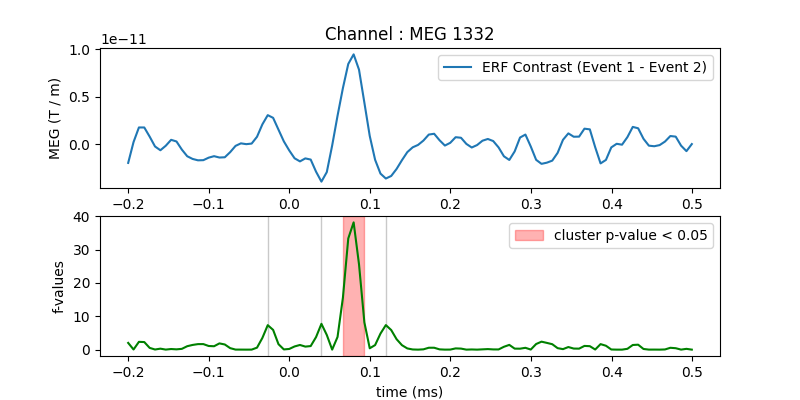# Permutation F-test on sensor data with 1D cluster level#

One tests if the evoked response is significantly different between conditions. Multiple comparison problem is addressed with cluster level permutation test.

```# Authors: Alexandre Gramfort <alexandre.gramfort@inria.fr>
#
```
```import matplotlib.pyplot as plt

import mne
from mne import io
from mne.stats import permutation_cluster_test
from mne.datasets import sample

print(__doc__)
```

Set parameters

```data_path = sample.data_path()
meg_path = data_path / "MEG" / "sample"
raw_fname = meg_path / "sample_audvis_filt-0-40_raw.fif"
event_fname = meg_path / "sample_audvis_filt-0-40_raw-eve.fif"
tmin = -0.2
tmax = 0.5

#   Setup for reading the raw data

channel = "MEG 1332"  # include only this channel in analysis
include = [channel]
```
```Opening raw data file /home/circleci/mne_data/MNE-sample-data/MEG/sample/sample_audvis_filt-0-40_raw.fif...
Read a total of 4 projection items:
PCA-v1 (1 x 102)  idle
PCA-v2 (1 x 102)  idle
PCA-v3 (1 x 102)  idle
Average EEG reference (1 x 60)  idle
Range : 6450 ... 48149 =     42.956 ...   320.665 secs
```

Read epochs for the channel of interest

```picks = mne.pick_types(raw.info, meg=False, eog=True, include=include, exclude="bads")
event_id = 1
epochs1 = mne.Epochs(
raw, events, event_id, tmin, tmax, picks=picks, baseline=(None, 0), reject=reject
)
condition1 = epochs1.get_data()  # as 3D matrix

event_id = 2
epochs2 = mne.Epochs(
raw, events, event_id, tmin, tmax, picks=picks, baseline=(None, 0), reject=reject
)
condition2 = epochs2.get_data()  # as 3D matrix

condition1 = condition1[:, 0, :]  # take only one channel to get a 2D array
condition2 = condition2[:, 0, :]  # take only one channel to get a 2D array
```
```Not setting metadata
72 matching events found
Setting baseline interval to [-0.19979521315838786, 0.0] s
Applying baseline correction (mode: mean)
4 projection items activated
Rejecting  epoch based on EOG : ['EOG 061']
Rejecting  epoch based on EOG : ['EOG 061']
Rejecting  epoch based on EOG : ['EOG 061']
Rejecting  epoch based on EOG : ['EOG 061']
Rejecting  epoch based on EOG : ['EOG 061']
Rejecting  epoch based on EOG : ['EOG 061']
Rejecting  epoch based on EOG : ['EOG 061']
Rejecting  epoch based on EOG : ['EOG 061']
Rejecting  epoch based on EOG : ['EOG 061']
Rejecting  epoch based on EOG : ['EOG 061']
Rejecting  epoch based on EOG : ['EOG 061']
Rejecting  epoch based on EOG : ['EOG 061']
Rejecting  epoch based on EOG : ['EOG 061']
Rejecting  epoch based on EOG : ['EOG 061']
Rejecting  epoch based on EOG : ['EOG 061']
Rejecting  epoch based on EOG : ['EOG 061']
73 matching events found
Setting baseline interval to [-0.19979521315838786, 0.0] s
Applying baseline correction (mode: mean)
4 projection items activated
Rejecting  epoch based on EOG : ['EOG 061']
Rejecting  epoch based on EOG : ['EOG 061']
Rejecting  epoch based on EOG : ['EOG 061']
Rejecting  epoch based on EOG : ['EOG 061']
Rejecting  epoch based on EOG : ['EOG 061']
Rejecting  epoch based on EOG : ['EOG 061']
Rejecting  epoch based on EOG : ['EOG 061']
Rejecting  epoch based on EOG : ['EOG 061']
Rejecting  epoch based on EOG : ['EOG 061']
Rejecting  epoch based on EOG : ['EOG 061']
Rejecting  epoch based on EOG : ['EOG 061']
```

Compute statistic

```threshold = 6.0
T_obs, clusters, cluster_p_values, H0 = permutation_cluster_test(
[condition1, condition2],
n_permutations=1000,
threshold=threshold,
tail=1,
n_jobs=None,
)
```
```stat_fun(H1): min=0.000227 max=38.167093
Running initial clustering …
Found 4 clusters

0%|          | Permuting : 0/999 [00:00<?,       ?it/s]
15%|█▍        | Permuting : 146/999 [00:00<00:00, 4235.41it/s]
30%|███       | Permuting : 301/999 [00:00<00:00, 4409.97it/s]
46%|████▌     | Permuting : 458/999 [00:00<00:00, 4490.99it/s]
62%|██████▏   | Permuting : 622/999 [00:00<00:00, 4586.35it/s]
79%|███████▉  | Permuting : 790/999 [00:00<00:00, 4665.11it/s]
95%|█████████▍| Permuting : 948/999 [00:00<00:00, 4663.33it/s]
100%|██████████| Permuting : 999/999 [00:00<00:00, 4631.13it/s]
```

Plot

```times = epochs1.times
fig, (ax, ax2) = plt.subplots(2, 1, figsize=(8, 4))
ax.set_title("Channel : " + channel)
ax.plot(
times,
condition1.mean(axis=0) - condition2.mean(axis=0),
label="ERF Contrast (Event 1 - Event 2)",
)
ax.set_ylabel("MEG (T / m)")
ax.legend()

for i_c, c in enumerate(clusters):
c = c
if cluster_p_values[i_c] <= 0.05:
h = ax2.axvspan(times[c.start], times[c.stop - 1], color="r", alpha=0.3)
else:
ax2.axvspan(times[c.start], times[c.stop - 1], color=(0.3, 0.3, 0.3), alpha=0.3)

hf = plt.plot(times, T_obs, "g")
ax2.legend((h,), ("cluster p-value < 0.05",))
ax2.set_xlabel("time (ms)")
ax2.set_ylabel("f-values")
```Total running time of the script: (0 minutes 4.730 seconds)

Estimated memory usage: 10 MB

Gallery generated by Sphinx-Gallery